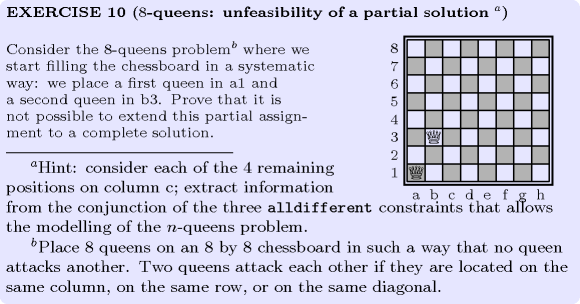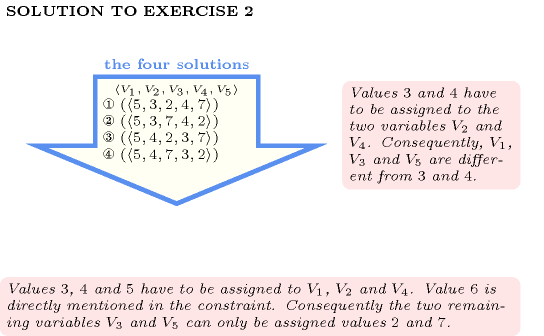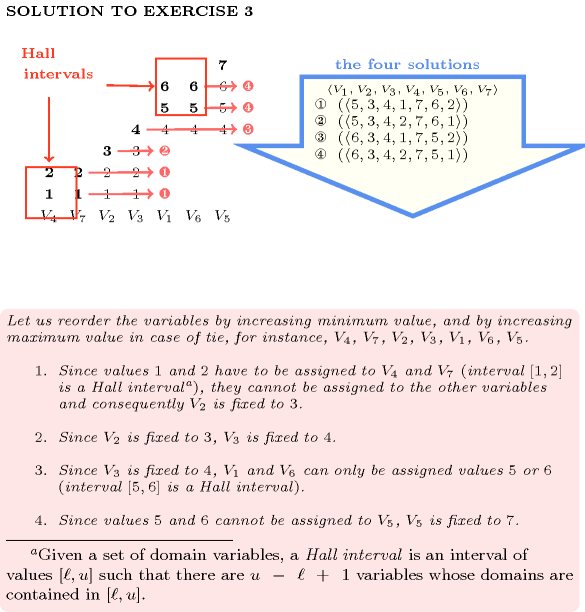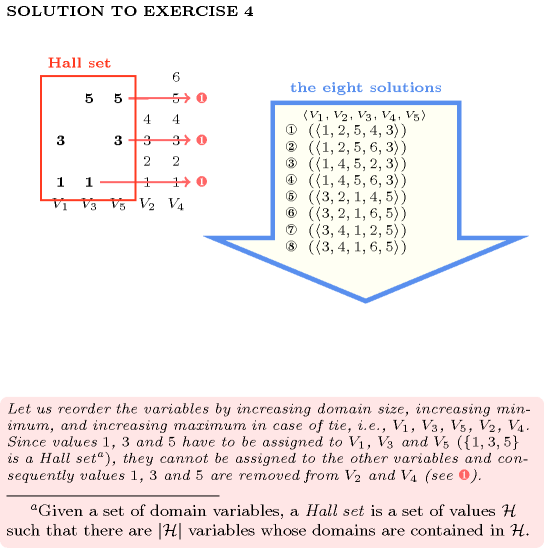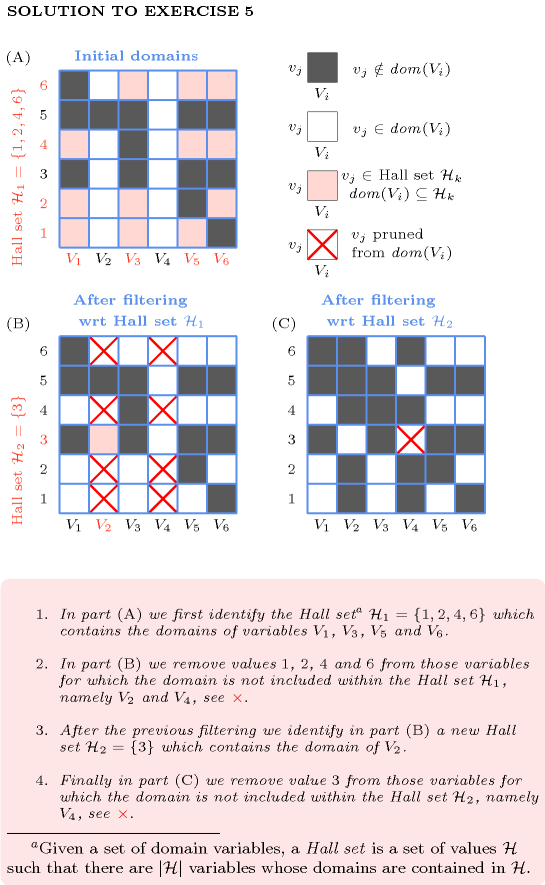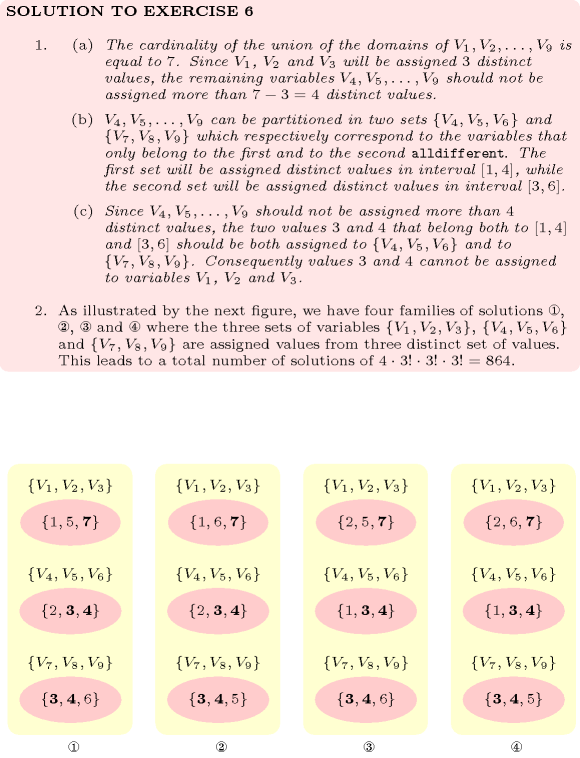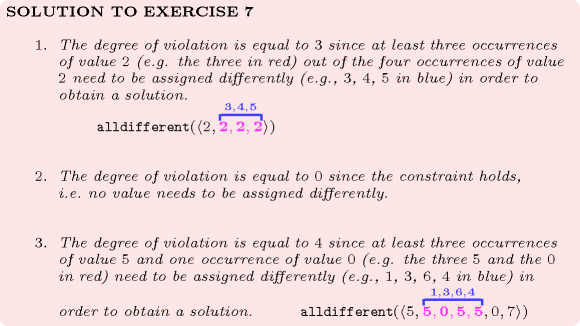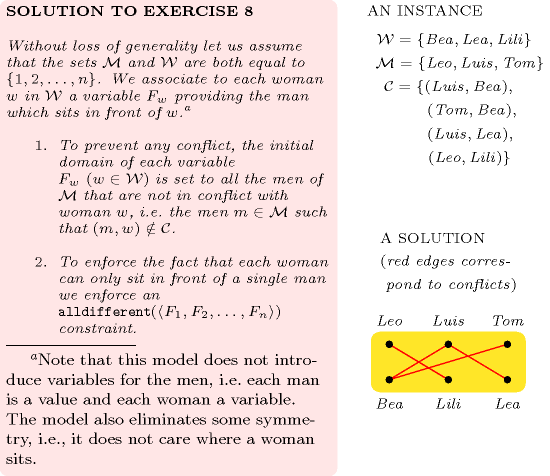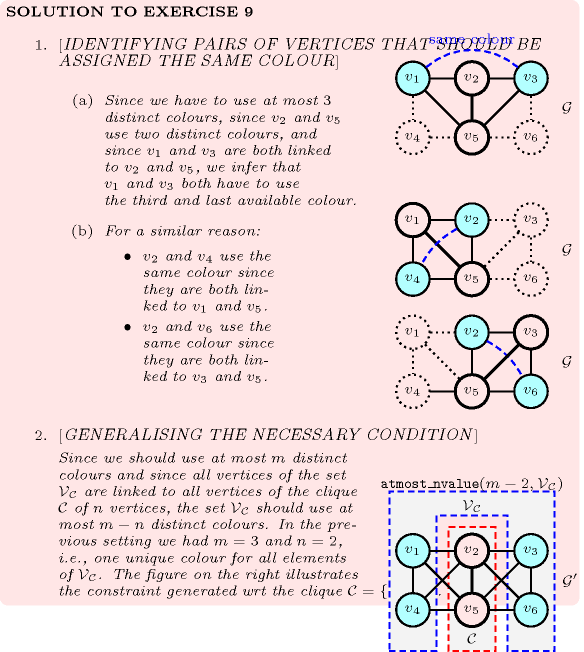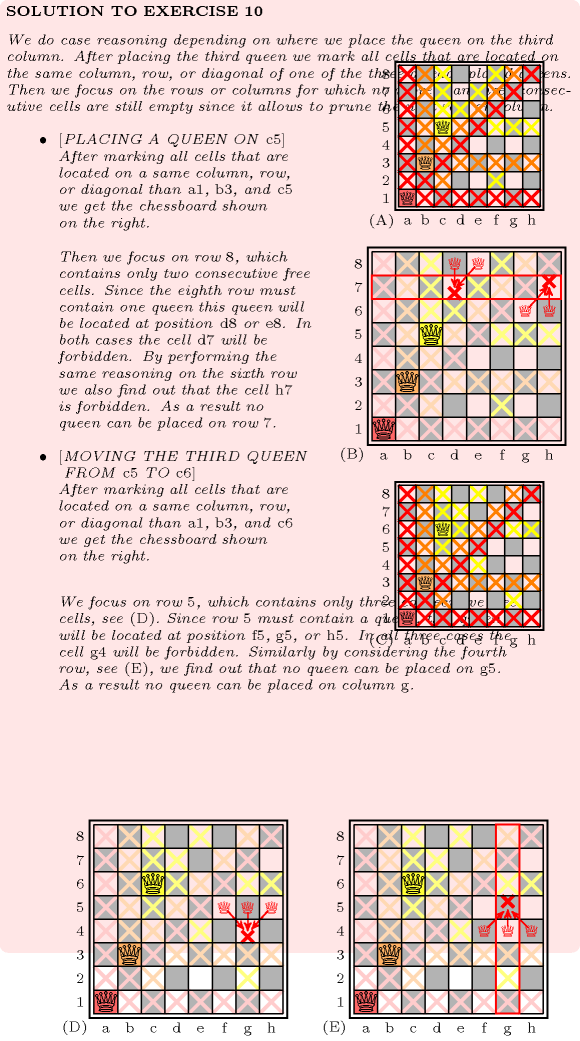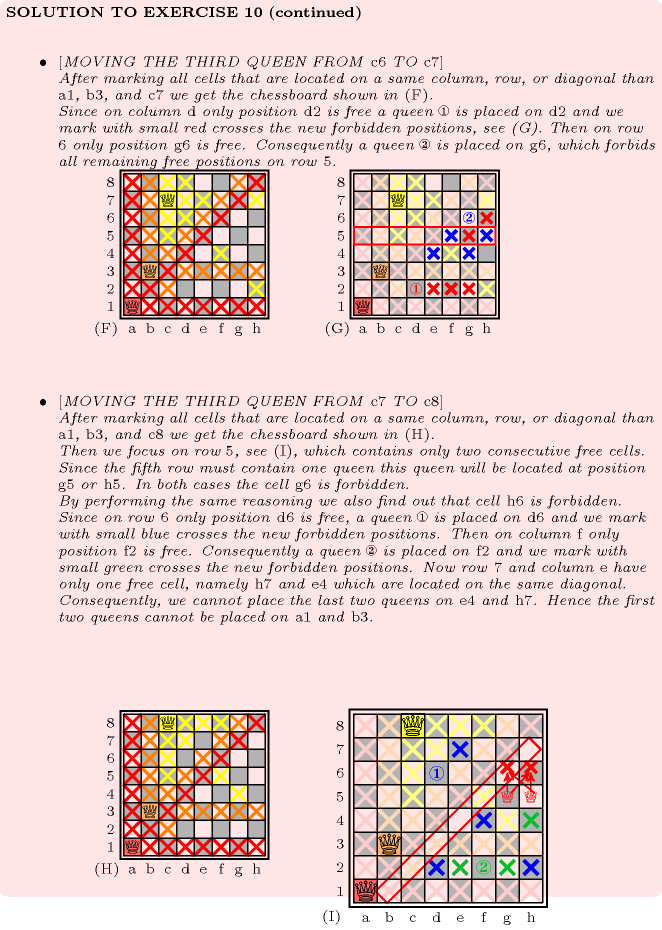## 5.12. alldifferent

Origin
Constraint

$\mathrm{𝚊𝚕𝚕𝚍𝚒𝚏𝚏𝚎𝚛𝚎𝚗𝚝}\left(\mathrm{𝚅𝙰𝚁𝙸𝙰𝙱𝙻𝙴𝚂}\right)$

Synonyms

$\mathrm{𝚊𝚕𝚕𝚍𝚒𝚏𝚏}$, $\mathrm{𝚊𝚕𝚕𝚍𝚒𝚜𝚝𝚒𝚗𝚌𝚝}$, $\mathrm{𝚍𝚒𝚜𝚝𝚒𝚗𝚌𝚝}$, $\mathrm{𝚋𝚘𝚞𝚗𝚍}_\mathrm{𝚊𝚕𝚕𝚍𝚒𝚏𝚏𝚎𝚛𝚎𝚗𝚝}$, $\mathrm{𝚋𝚘𝚞𝚗𝚍}_\mathrm{𝚊𝚕𝚕𝚍𝚒𝚏𝚏}$, $\mathrm{𝚋𝚘𝚞𝚗𝚍}_\mathrm{𝚍𝚒𝚜𝚝𝚒𝚗𝚌𝚝}$, $\mathrm{𝚛𝚎𝚕}$.

Argument
 $\mathrm{𝚅𝙰𝚁𝙸𝙰𝙱𝙻𝙴𝚂}$ $\mathrm{𝚌𝚘𝚕𝚕𝚎𝚌𝚝𝚒𝚘𝚗}\left(\mathrm{𝚟𝚊𝚛}-\mathrm{𝚍𝚟𝚊𝚛}\right)$
Restriction
$\mathrm{𝚛𝚎𝚚𝚞𝚒𝚛𝚎𝚍}$$\left(\mathrm{𝚅𝙰𝚁𝙸𝙰𝙱𝙻𝙴𝚂},\mathrm{𝚟𝚊𝚛}\right)$
Purpose

Enforce all variables of the collection $\mathrm{𝚅𝙰𝚁𝙸𝙰𝙱𝙻𝙴𝚂}$ to take distinct values.

Example
$\left(〈5,1,9,3〉\right)$

The $\mathrm{𝚊𝚕𝚕𝚍𝚒𝚏𝚏𝚎𝚛𝚎𝚗𝚝}$ constraint holds since all the values 5, 1, 9 and 3 are distinct.

All solutions

Figure 5.12.1 gives all solutions to the following non ground instance of the $\mathrm{𝚊𝚕𝚕𝚍𝚒𝚏𝚏𝚎𝚛𝚎𝚗𝚝}$ constraint: ${V}_{1}\in \left[2,4\right]$, ${V}_{2}\in \left[2,3\right]$, ${V}_{3}\in \left[1,6\right]$, ${V}_{4}\in \left[2,5\right]$, ${V}_{5}\in \left[2,3\right]$, ${V}_{6}\in \left[1,6\right]$, $\mathrm{𝚊𝚕𝚕𝚍𝚒𝚏𝚏𝚎𝚛𝚎𝚗𝚝}$$\left(〈{V}_{1},{V}_{2},{V}_{3},{V}_{4},{V}_{5},{V}_{6}〉\right)$.

##### Figure 5.12.1. All solutions corresponding to the non ground example of the $\mathrm{𝚊𝚕𝚕𝚍𝚒𝚏𝚏𝚎𝚛𝚎𝚗𝚝}$ constraint of the All solutions slotTypical
$|\mathrm{𝚅𝙰𝚁𝙸𝙰𝙱𝙻𝙴𝚂}|>2$
Symmetries
• Items of $\mathrm{𝚅𝙰𝚁𝙸𝙰𝙱𝙻𝙴𝚂}$ are permutable.

• Two distinct values of $\mathrm{𝚅𝙰𝚁𝙸𝙰𝙱𝙻𝙴𝚂}.\mathrm{𝚟𝚊𝚛}$ can be swapped; a value of $\mathrm{𝚅𝙰𝚁𝙸𝙰𝙱𝙻𝙴𝚂}.\mathrm{𝚟𝚊𝚛}$ can be renamed to any unused value.

Arg. properties

Contractible wrt. $\mathrm{𝚅𝙰𝚁𝙸𝙰𝙱𝙻𝙴𝚂}$.

Usage

The $\mathrm{𝚊𝚕𝚕𝚍𝚒𝚏𝚏𝚎𝚛𝚎𝚗𝚝}$ constraint occurs in most practical problems directly or indirectly. A classical example is the n-queens chess puzzle problem: Place $n$ queens on an $n$ by $n$ chessboard in such a way that no queen attacks another. Two queens attack each other if they are located on the same column, on the same row, or on the same diagonal. This can be modelled as the conjunction of three $\mathrm{𝚊𝚕𝚕𝚍𝚒𝚏𝚏𝚎𝚛𝚎𝚗𝚝}$ constraints. We associate to column $i$ of the chessboard a domain variable ${X}_{i}$ that gives the row number where the corresponding queen is located. The three $\mathrm{𝚊𝚕𝚕𝚍𝚒𝚏𝚏𝚎𝚛𝚎𝚗𝚝}$ constraints are:

• $\mathrm{𝚊𝚕𝚕𝚍𝚒𝚏𝚏𝚎𝚛𝚎𝚗𝚝}\left({X}_{1},{X}_{2}+1,\cdots ,{X}_{n}+n-1\right)$ for the descending diagonals,

• $\mathrm{𝚊𝚕𝚕𝚍𝚒𝚏𝚏𝚎𝚛𝚎𝚗𝚝}\left({X}_{1},{X}_{2},\cdots ,{X}_{n}\right)$ for the rows,

• $\mathrm{𝚊𝚕𝚕𝚍𝚒𝚏𝚏𝚎𝚛𝚎𝚗𝚝}\left({X}_{1}+n-1,{X}_{2}+n-2,\cdots ,{X}_{n}\right)$ for the ascending diagonals.

They are respectively depicted by parts (A), (C) and (D) of Figure 5.12.2. Figure 5.12.3 makes explicit the link between the two families of diagonals and the corresponding $\mathrm{𝚊𝚕𝚕𝚍𝚒𝚏𝚏𝚎𝚛𝚎𝚗𝚝}$ constraints. Note that this model matches the checker introduced by Gauss to test whether a permutation of row numbers is a solution or not to the 8 queens problem: first add the numbers 1 up to 8 to the permutation and check that the resulting numbers are distinct, second add the numbers 8 down to 1 and perform the same check [Watkins04].

##### Figure 5.12.2. Descending diagonals (A-B), rows (C) and ascending diagonals (D-E)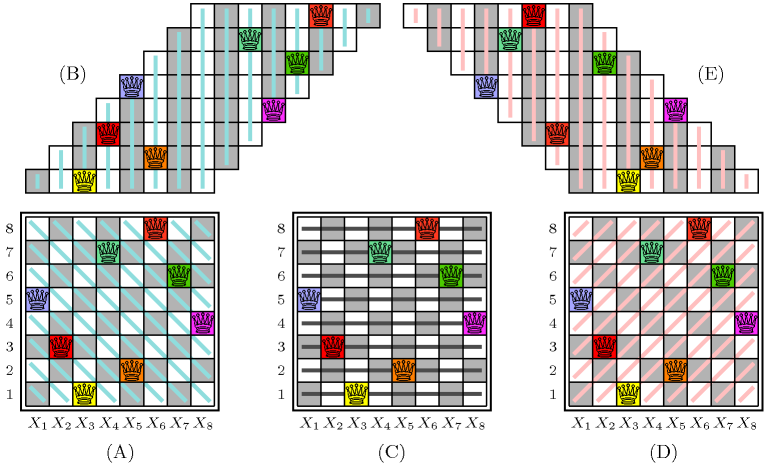##### Figure 5.12.3. (A) For every pair of columns $i$, $j$ ($i), given the position ${X}_{i}$ of the queen on column $i$, we respectively have from the ascending and descending diagonals that ${X}_{j}\ne {X}_{i}+\left(j-i\right)$ and ${X}_{j}\ne {X}_{i}-\left(j-i\right)$ (B) Equivalence of the two $\mathrm{𝚊𝚕𝚕𝚍𝚒𝚏𝚏𝚎𝚛𝚎𝚗𝚝}$ constraints respectively associated with the ascending and descending diagonals with the two families of disequalities (i.e., the orange and the red one) depicted in Part (A)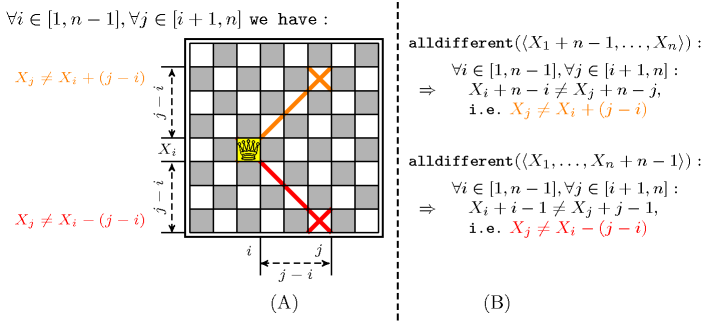A second example taken from [AsratianDenleyHaggkvist98], where the bipartite graph associated with the $\mathrm{𝚊𝚕𝚕𝚍𝚒𝚏𝚏𝚎𝚛𝚎𝚗𝚝}$ constraint is convex, is a ski assignment problem: “a set of skiers have each specified the smallest and largest ski sizes they will accept from a given set of ski sizes”. The task is to find a ski size for each skier.

Examples such as Costas arrays and Golomb rulers involve one or several $\mathrm{𝚊𝚕𝚕𝚍𝚒𝚏𝚏𝚎𝚛𝚎𝚗𝚝}$ constraints on differences of variables.

Quite often, the $\mathrm{𝚊𝚕𝚕𝚍𝚒𝚏𝚏𝚎𝚛𝚎𝚗𝚝}$ constraint is also used in conjunction with several $\mathrm{𝚎𝚕𝚎𝚖𝚎𝚗𝚝}$ constraints, especially in the context of assignment problems [Hooker07book], or with several precedence constraints, especially in the context of symmetry breaking or scheduling problems [BessiereNarodytskaQuimperWalsh11].

Other examples involving several $\mathrm{𝚊𝚕𝚕𝚍𝚒𝚏𝚏𝚎𝚛𝚎𝚗𝚝}$ constraints sharing some variables can be found in the Usage slot of the $𝚔_\mathrm{𝚊𝚕𝚕𝚍𝚒𝚏𝚏𝚎𝚛𝚎𝚗𝚝}$ constraint.

Remark

Even if the $\mathrm{𝚊𝚕𝚕𝚍𝚒𝚏𝚏𝚎𝚛𝚎𝚗𝚝}$ constraint did not have this form, it was specified in ALICE [Lauriere76], [Lauriere78] by asking for an injective correspondence $f$ between variables and values: $x\ne y⇒f\left(x\right)\ne f\left(y\right)$. From an algorithmic point of view, the algorithm for computing the cardinality of the maximum matching of a bipartite graph was not used in ALICE for checking the feasibility of the $\mathrm{𝚊𝚕𝚕𝚍𝚒𝚏𝚏𝚎𝚛𝚎𝚗𝚝}$ constraint, even though the algorithm was already known in 1976. This is because the goal of ALICE was to show that a general system could be as efficient as dedicated algorithms. For this reason the concluding part of [Lauriere76] explicitly mentions that specialised algorithms should be discarded. On the one hand, many people, especially from the OR community, have complained about such a radical statement [Roy06]. On the other hand, the motivation of such a statement stands from the fact that a truly intelligent system should not rely on black-box algorithms, but should rather be able to reconstruct them from some kind of first principles. How to achieve this is still an open question.

Some solvers use, in a pre-processing phase before stating all constraints, an algorithm for automatically extracting large cliques [BronKerbosch73], [EppsteinStrash11] from a set of binary disequalities in order to replace them by $\mathrm{𝚊𝚕𝚕𝚍𝚒𝚏𝚏𝚎𝚛𝚎𝚗𝚝}$ constraints.

W.-J. van Hoeve provides a survey about the $\mathrm{𝚊𝚕𝚕𝚍𝚒𝚏𝚏𝚎𝚛𝚎𝚗𝚝}$ constraint in [Hoeve01].

For possible relaxation of the $\mathrm{𝚊𝚕𝚕𝚍𝚒𝚏𝚏𝚎𝚛𝚎𝚗𝚝}$ constraints see the $\mathrm{𝚊𝚕𝚕𝚍𝚒𝚏𝚏𝚎𝚛𝚎𝚗𝚝}_\mathrm{𝚎𝚡𝚌𝚎𝚙𝚝}_\mathtt{0}$, the $𝚔_\mathrm{𝚊𝚕𝚕𝚍𝚒𝚏𝚏𝚎𝚛𝚎𝚗𝚝}$ (i.e., $\mathrm{𝚜𝚘𝚖𝚎}_\mathrm{𝚍𝚒𝚏𝚏𝚎𝚛𝚎𝚗𝚝}$

Within the context of linear programming, relaxations of the $\mathrm{𝚊𝚕𝚕𝚍𝚒𝚏𝚏𝚎𝚛𝚎𝚗𝚝}$ constraint are described in [WilliamsYan01] and in [Hooker07book].

Within the context of constraint-centered search heuristics, G. Pesant and A. Zanarini [ZanariniPesant07b] have proposed several estimators for evaluating the number of solutions of an $\mathrm{𝚊𝚕𝚕𝚍𝚒𝚏𝚏𝚎𝚛𝚎𝚗𝚝}$ constraint (since counting the total number of maximum matchings of the corresponding variable-value graph is #P-complete [Valiant79]). Faster, but less accurate estimators, based on upper bounds of the number of solutions were proposed three years later by the same authors [ZanariniPesant10].

Given $n$ variables taking their values within the interval $\left[1,n\right]$, the total number of solutions to the corresponding $\mathrm{𝚊𝚕𝚕𝚍𝚒𝚏𝚏𝚎𝚛𝚎𝚗𝚝}$ constraint corresponds to the sequence A000142 of the On-Line Encyclopaedia of Integer Sequences [Sloane10].

Algorithm

The first complete filtering algorithm was independently found by M.-C. Costa [Costa94] and J.-C. Régin [Regin94]. This algorithm is based on a corollary of C. Berge that characterises the edges of a graph that belong to a maximum matching but not to all [Berge70].A similar result is in fact given in [Petersen1891]. Similarly, Dulmage-Mendelsohn decomposition [DulmageMendelsohn58] was also used recently by [Cymer12], [CymerPhD13] to characterise such edges and prune the corresponding variables both for the $\mathrm{𝚊𝚕𝚕𝚍𝚒𝚏𝚏𝚎𝚛𝚎𝚗𝚝}$ constraint and for other constraints like $\mathrm{𝚊𝚕𝚕𝚍𝚒𝚏𝚏𝚎𝚛𝚎𝚗𝚝}_\mathrm{𝚎𝚡𝚌𝚎𝚙𝚝}_\mathtt{0}$, $\mathrm{𝚌𝚘𝚛𝚛𝚎𝚜𝚙𝚘𝚗𝚍𝚎𝚗𝚌𝚎}$, $\mathrm{𝚒𝚗𝚟𝚎𝚛𝚜𝚎}$, $\mathrm{𝚜𝚊𝚖𝚎}$, $\mathrm{𝚞𝚜𝚎𝚍}_\mathrm{𝚋𝚢}$, $\mathrm{𝚐𝚕𝚘𝚋𝚊𝚕}_\mathrm{𝚌𝚊𝚛𝚍𝚒𝚗𝚊𝚕𝚒𝚝𝚢}_\mathrm{𝚕𝚘𝚠}_\mathrm{𝚞𝚙}$, $\mathrm{𝚜𝚘𝚏𝚝}_\mathrm{𝚊𝚕𝚕𝚍𝚒𝚏𝚏𝚎𝚛𝚎𝚗𝚝}_\mathrm{𝚟𝚊𝚛}$, $\mathrm{𝚜𝚘𝚏𝚝}_\mathrm{𝚜𝚊𝚖𝚎}_\mathrm{𝚟𝚊𝚛}$, $\mathrm{𝚜𝚘𝚏𝚝}_\mathrm{𝚞𝚜𝚎𝚍}_\mathrm{𝚋𝚢}_\mathrm{𝚟𝚊𝚛}$. Assuming that all variables have no holes in their domain, M. Leconte came up with a filtering algorithm [Leconte96] based on edge finding. A first bound-consistency algorithm was proposed by Bleuzen-Guernalec et al. [GuernalecColmerauer97]. Later on, two different approaches were used to design bound-consistency algorithms. Both approaches model the constraint as a bipartite graph. The first identifies Hall intervals in this graph [Puget98], [LopezOrtizQuimperTrompBeek03] and the second applies the same algorithm that is used to compute arc-consistency, but achieves a speedup by exploiting the simpler structure [Glover67] of the graph [MehlhornThiel00]. Ian P. Gent et al. discuss in [GentMiguelNightingale08] implementations issues behind the complete filtering algorithm and in particular the computation of the strongly connected components of the residual graph (i.e., a graph constructed from a maximum variable-value matching and from the possible values of the variables of the $\mathrm{𝚊𝚕𝚕𝚍𝚒𝚏𝚏𝚎𝚛𝚎𝚗𝚝}$ constraint), which appears to be the main bottleneck in practice. Figures 2.1.1 and 2.1.2 of Section 2.1.3 illustrate the filtering of the $\mathrm{𝚊𝚕𝚕𝚍𝚒𝚏𝚏𝚎𝚛𝚎𝚗𝚝}$ constraint with respect to arc-consistency and bound-consistency. The leftmost part of Figure 3.7.28 illustrates a flow model for the $\mathrm{𝚊𝚕𝚕𝚍𝚒𝚏𝚏𝚎𝚛𝚎𝚗𝚝}$ constraint where there is a one-to-one correspondence between feasible flows in the flow model and solutions of the $\mathrm{𝚊𝚕𝚕𝚍𝚒𝚏𝚏𝚎𝚛𝚎𝚗𝚝}$ constraint.

From a worst case complexity point of view, assuming that $n$ is the number of variables and $m$ the sum of the domains sizes, we have the following complexity results:

• Complete filtering is achieved in $O\left(m\sqrt{n}\right)$ by Régin's algorithm [Regin94].

• Range consistency is done in $O\left({n}^{2}\right)$ by Leconte's algorithm [Leconte96].

• Bound-consistency is performed in $O\left(nlogn\right)$ in [Puget98], [MehlhornThiel00], [LopezOrtizQuimperTrompBeek03]. If sort can be achieved in linear time, typically when the $\mathrm{𝚊𝚕𝚕𝚍𝚒𝚏𝚏𝚎𝚛𝚎𝚗𝚝}$ constraint encodes a permutation,In this context the total number of values that can be assigned to the variables of the $\mathrm{𝚊𝚕𝚕𝚍𝚒𝚏𝚏𝚎𝚛𝚎𝚗𝚝}$ constraint is equal to the number of variables. Under this assumption sorting the variables on their minimum or maximum values can be achieved in linear time. the worst case complexity of the algorithms described in [MehlhornThiel00], [LopezOrtizQuimperTrompBeek03] goes down to $O\left(n\right)$.

Within the context of explanations [JussienBarichard00], the explanation of the filtering algorithm that achieves arc-consistency for the $\mathrm{𝚊𝚕𝚕𝚍𝚒𝚏𝚏𝚎𝚛𝚎𝚗𝚝}$ constraint is described in [Rochart05]. Given the residual graph (i.e., a graph constructed from a maximum variable-value matching and from the possible values of the variables of the $\mathrm{𝚊𝚕𝚕𝚍𝚒𝚏𝚏𝚎𝚛𝚎𝚗𝚝}$ constraint), the removal of an arc starting from a vertex belonging to a strongly connected component ${𝒞}_{1}$ to a distinct strongly connected component ${𝒞}_{2}$ is explained by all missing arcs starting from a descendant component of ${𝒞}_{2}$ and ending in an ancestor component of ${𝒞}_{1}$ (i.e., since the addition of any of these missing arcs would merge the strongly connected components ${𝒞}_{1}$ and ${𝒞}_{2}$). Let us illustrate this on a concrete example. For this purpose assume we have the following variables and the values that can potentially be assigned to each of them, $A\in \left\{1,2\right\}$, $B\in \left\{1,2\right\}$, $C\in \left\{2,3,4,6\right\}$, $D\in \left\{3,4\right\}$, $E\in \left\{5,6\right\}$, $F\in \left\{5,6\right\}$, $G\in \left\{6,7,8\right\}$, $H\in \left\{6,7,8\right\}$. Figure 5.12.4 represents the residual graph associated with the maximum matching corresponding to the assignment $A=1$, $B=2$, $C=3$, $D=4$, $E=5$, $F=6$, $G=7$, $H=8$. It has four strongly connected components containing respectively vertices $\left\{A,B,1,2\right\}$, $\left\{C,D,3,4\right\}$, $\left\{E,F,5,6\right\}$ and $\left\{G,H,7,8\right\}$. Arcs that are between strongly connected components correspond to values that can be removed:

• The removal of value 2 from variable $C$ is explained by the absence of the arcs corresponding to the assignments $A=3$, $A=4$, $B=3$ and $B=4$ (since adding any of these missing arcs would merge the blue and the pink strongly connected components containing the vertices corresponding to value 2 and variable $C$).

• The removal of value 6 from variable $C$ is explained by the absence of the arcs corresponding to the assignments $E=3$, $E=4$, $F=3$ and $F=4$. Again adding the corresponding arcs would merge the two strongly connected components containing the vertices corresponding to value 6 and variable $C$.

• The removal of value 6 from variable $G$ is explained by the absence of the arcs corresponding to the assignments $E=7$, $E=8$, $F=7$ and $F=8$.

• The removal of value 6 from variable $H$ is explained by the absence of the arcs corresponding to the assignments $E=7$, $E=8$, $F=7$ and $F=8$.

##### Figure 5.12.4. Strongly connected components of the residual graph illustrating the explanation of the removal of a value for the constraint $\mathrm{𝚊𝚕𝚕𝚍𝚒𝚏𝚏𝚎𝚛𝚎𝚗𝚝}$$\left(〈A,B,C,D,E,F,G,H〉\right)$, $A\in \left\{1,2\right\}$, $B\in \left\{1,2\right\}$, $C\in \left\{2,3,4,6\right\}$, $D\in \left\{3,4\right\}$, $E\in \left\{5,6\right\}$, $F\in \left\{5,6\right\}$, $G\in \left\{6,7,8\right\}$, $H\in \left\{6,7,8\right\}$: the explanation why value 2 is removed from variable $C$ corresponds to all missing arcs whose addition would merge the blue and the pink strongly connected components (i.e., the missing arcs corresponding to the assignments $A=3$, $A=4$, $B=3$ and $B=4$ that are depicted by thick pink lines)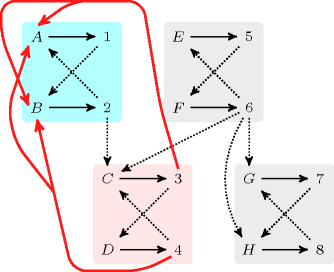An additional example for illustrating the generation of explanations for the $\mathrm{𝚊𝚕𝚕𝚍𝚒𝚏𝚏𝚎𝚛𝚎𝚗𝚝}$ constraint when there are more values than variables is provided by Figure 2.1.3 of Section 2.1.4.

After applying bound-consistency the following property holds for all variables of an $\mathrm{𝚊𝚕𝚕𝚍𝚒𝚏𝚏𝚎𝚛𝚎𝚗𝚝}$ constraint. Given a Hall interval $\left[l,u\right]$, any variable $V$ whose range $\left[\underline{V},\overline{V}\right]$ intersects $\left[l,u\right]$ without being included in $\left[l,u\right]$ has its minimum value $\underline{V}$ (respectively maximum value $\overline{V}$) that is located before (respectively after) the Hall interval (i.e., $\underline{V}).

The $\mathrm{𝚊𝚕𝚕𝚍𝚒𝚏𝚏𝚎𝚛𝚎𝚗𝚝}$ constraint is entailed if and only if there is no value $v$ that can be assigned two distinct variables of the $\mathrm{𝚅𝙰𝚁𝙸𝙰𝙱𝙻𝙴𝚂}$ collection (i.e., the intersection of the two sets of potential values of any pair of variables is empty).

Reformulation

The $\mathrm{𝚊𝚕𝚕𝚍𝚒𝚏𝚏𝚎𝚛𝚎𝚗𝚝}$ constraint can be reformulated into a set of disequalities constraints. This model neither preserves bound-consistency nor arc-consistency:

• On the one hand a model, involving linear constraints, preserving bound-consistency was introduced in [BessiereKatsirelosNarodytskaQuimperWalsh09IJCAI]. For each potential interval $\left[l,u\right]$ of consecutive values this model uses $|\mathrm{𝚅𝙰𝚁𝙸𝙰𝙱𝙻𝙴𝚂}|$ 0-1 variables ${B}_{1,l,u},{B}_{2,l,u},\cdots ,{B}_{|\mathrm{𝚅𝙰𝚁𝙸𝙰𝙱𝙻𝙴𝚂}|,l,u}$ for modelling that each variable of the collection $\mathrm{𝚅𝙰𝚁𝙸𝙰𝙱𝙻𝙴𝚂}$ is assigned a value within interval $\left[l,u\right]$ (i.e., $\forall i\in \left[1,|\mathrm{𝚅𝙰𝚁𝙸𝙰𝙱𝙻𝙴𝚂}|\right]:{B}_{i,l,u}⇔\mathrm{𝚅𝙰𝚁𝙸𝙰𝙱𝙻𝙴𝚂}\left[i\right].\mathrm{𝚟𝚊𝚛}\in \left[l,u\right]$),How to encode the reified constraint ${B}_{i,l,u}⇔\mathrm{𝚅𝙰𝚁𝙸𝙰𝙱𝙻𝙴𝚂}\left[i\right].\mathrm{𝚟𝚊𝚛}\in \left[l,u\right]$ with linear constraints is described in the Reformulation slot of the $\mathrm{𝚒𝚗}_\mathrm{𝚒𝚗𝚝𝚎𝚛𝚟𝚊𝚕}_\mathrm{𝚛𝚎𝚒𝚏𝚒𝚎𝚍}$ constraint. and an inequality constraint for enforcing the condition that the sum of the corresponding 0-1 variables is less than or equal to the size $u-l+1$ of the corresponding interval (i.e. ${B}_{1,l,u}+{B}_{2,l,u}+\cdots +{B}_{|\mathrm{𝚅𝙰𝚁𝙸𝙰𝙱𝙻𝙴𝚂}|,l,u}\le u-l+1$).

• On the other hand, it was shown in [BessiereKatsirelosNarodytskaWalsh09] that there is no polynomial sized decomposition that preserves arc-consistency.

Finally the $\mathrm{𝚊𝚕𝚕𝚍𝚒𝚏𝚏𝚎𝚛𝚎𝚗𝚝}$$\left(\mathrm{𝚅𝙰𝚁𝙸𝙰𝙱𝙻𝙴𝚂}\right)$ constraint can also be reformulated as the conjunction $\mathrm{𝚜𝚘𝚛𝚝}$$\left(\mathrm{𝚅𝙰𝚁𝙸𝙰𝙱𝙻𝙴𝚂},\mathrm{𝚂𝙾𝚁𝚃𝙴𝙳}_\mathrm{𝚅𝙰𝚁𝙸𝙰𝙱𝙻𝙴𝚂}\right)\wedge$ $\mathrm{𝚜𝚝𝚛𝚒𝚌𝚝𝚕𝚢}_\mathrm{𝚒𝚗𝚌𝚛𝚎𝚊𝚜𝚒𝚗𝚐}$$\left(\mathrm{𝚂𝙾𝚁𝚃𝙴𝙳}_\mathrm{𝚅𝙰𝚁𝙸𝙰𝙱𝙻𝙴𝚂}\right)$. Unlike the naive reformulation, i.e., a $\mathrm{𝚍𝚒𝚜𝚎𝚚𝚞𝚊𝚕𝚒𝚝𝚢}$ constraint between each pair of variables, the $\mathrm{𝚜𝚘𝚛𝚝}$-based reformulation is linear in space.

Counting
 Length ($n$) 2 3 4 5 6 7 8 9 10 Solutions 6 24 120 720 5040 40320 362880 3628800 39916800

Number of solutions for $\mathrm{𝚊𝚕𝚕𝚍𝚒𝚏𝚏𝚎𝚛𝚎𝚗𝚝}$: domains $0..n$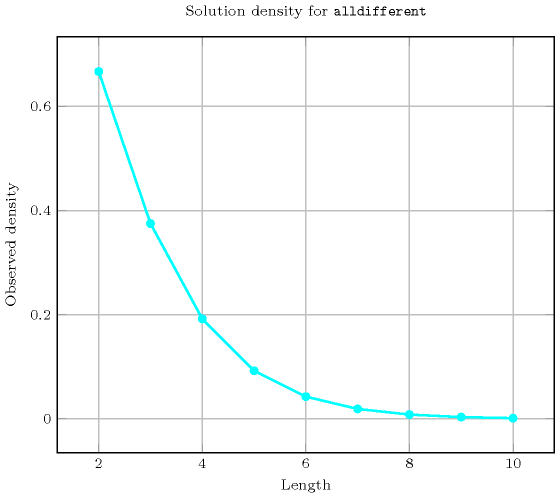Systems
Used in

generalisation: $\mathrm{𝚊𝚕𝚕}_\mathrm{𝚖𝚒𝚗}_\mathrm{𝚍𝚒𝚜𝚝}$ ($\mathrm{𝚟𝚊𝚛𝚒𝚊𝚋𝚕𝚎}$ replaced by $\mathrm{𝚕𝚒𝚗𝚎}\mathrm{𝚜𝚎𝚐𝚖𝚎𝚗𝚝}$, all of the same size), $\mathrm{𝚊𝚕𝚕𝚍𝚒𝚏𝚏𝚎𝚛𝚎𝚗𝚝}_\mathrm{𝚋𝚎𝚝𝚠𝚎𝚎𝚗}_\mathrm{𝚜𝚎𝚝𝚜}$ ($\mathrm{𝚟𝚊𝚛𝚒𝚊𝚋𝚕𝚎}$ replaced by $\mathrm{𝚜𝚎𝚝}\mathrm{𝚟𝚊𝚛𝚒𝚊𝚋𝚕𝚎}$), $\mathrm{𝚊𝚕𝚕𝚍𝚒𝚏𝚏𝚎𝚛𝚎𝚗𝚝}_\mathrm{𝚌𝚜𝚝}$ ($\mathrm{𝚟𝚊𝚛𝚒𝚊𝚋𝚕𝚎}$ replaced by $\mathrm{𝚟𝚊𝚛𝚒𝚊𝚋𝚕𝚎}+\mathrm{𝚌𝚘𝚗𝚜𝚝𝚊𝚗𝚝}$), $\mathrm{𝚊𝚕𝚕𝚍𝚒𝚏𝚏𝚎𝚛𝚎𝚗𝚝}_\mathrm{𝚒𝚗𝚝𝚎𝚛𝚟𝚊𝚕}$ ($\mathrm{𝚟𝚊𝚛𝚒𝚊𝚋𝚕𝚎}$ replaced by $\mathrm{𝚟𝚊𝚛𝚒𝚊𝚋𝚕𝚎}/\mathrm{𝚌𝚘𝚗𝚜𝚝𝚊𝚗𝚝}$), $\mathrm{𝚊𝚕𝚕𝚍𝚒𝚏𝚏𝚎𝚛𝚎𝚗𝚝}_\mathrm{𝚖𝚘𝚍𝚞𝚕𝚘}$ ($\mathrm{𝚟𝚊𝚛𝚒𝚊𝚋𝚕𝚎}$ replaced by $\mathrm{𝚟𝚊𝚛𝚒𝚊𝚋𝚕𝚎}\mathrm{mod}\mathrm{𝚌𝚘𝚗𝚜𝚝𝚊𝚗𝚝}$), $\mathrm{𝚊𝚕𝚕𝚍𝚒𝚏𝚏𝚎𝚛𝚎𝚗𝚝}_\mathrm{𝚙𝚊𝚛𝚝𝚒𝚝𝚒𝚘𝚗}$ ($\mathrm{𝚟𝚊𝚛𝚒𝚊𝚋𝚕𝚎}$ replaced by $\mathrm{𝚟𝚊𝚛𝚒𝚊𝚋𝚕𝚎}\in \mathrm{𝚙𝚊𝚛𝚝𝚒𝚝𝚒𝚘𝚗}$), $\mathrm{𝚍𝚒𝚏𝚏𝚗}$ ($\mathrm{𝚟𝚊𝚛𝚒𝚊𝚋𝚕𝚎}$ replaced by orthotope), $\mathrm{𝚍𝚒𝚜𝚓𝚞𝚗𝚌𝚝𝚒𝚟𝚎}$ ($\mathrm{𝚟𝚊𝚛𝚒𝚊𝚋𝚕𝚎}$ replaced by $\mathrm{𝚝𝚊𝚜𝚔}$), $\mathrm{𝚐𝚕𝚘𝚋𝚊𝚕}_\mathrm{𝚌𝚊𝚛𝚍𝚒𝚗𝚊𝚕𝚒𝚝𝚢}$ (control the number of occurrence of each value with a counter variable), $\mathrm{𝚐𝚕𝚘𝚋𝚊𝚕}_\mathrm{𝚌𝚊𝚛𝚍𝚒𝚗𝚊𝚕𝚒𝚝𝚢}_\mathrm{𝚕𝚘𝚠}_\mathrm{𝚞𝚙}$ (control the number of occurrence of each value with an interval), $\mathrm{𝚕𝚎𝚡}_\mathrm{𝚊𝚕𝚕𝚍𝚒𝚏𝚏𝚎𝚛𝚎𝚗𝚝}$ ($\mathrm{𝚟𝚊𝚛𝚒𝚊𝚋𝚕𝚎}$ replaced by $\mathrm{𝚟𝚎𝚌𝚝𝚘𝚛}$), $\mathrm{𝚗𝚟𝚊𝚕𝚞𝚎}$ (count number of distinct values).

Keywords
Cond. implications

$•$ $\mathrm{𝚊𝚕𝚕𝚍𝚒𝚏𝚏𝚎𝚛𝚎𝚗𝚝}\left(\mathrm{𝚅𝙰𝚁𝙸𝙰𝙱𝙻𝙴𝚂}\right)$

implies $\mathrm{𝚕𝚎𝚡}_\mathrm{𝚊𝚕𝚕𝚍𝚒𝚏𝚏𝚎𝚛𝚎𝚗𝚝}$$\left(\mathrm{𝚅𝙴𝙲𝚃𝙾𝚁𝚂}:\mathrm{𝚅𝙰𝚁𝙸𝙰𝙱𝙻𝙴𝚂}\right)$.

$•$ $\mathrm{𝚊𝚕𝚕𝚍𝚒𝚏𝚏𝚎𝚛𝚎𝚗𝚝}\left(\mathrm{𝚅𝙰𝚁𝙸𝙰𝙱𝙻𝙴𝚂}\right)$

implies $\mathrm{𝚜𝚘𝚏𝚝}_\mathrm{𝚊𝚕𝚕𝚍𝚒𝚏𝚏𝚎𝚛𝚎𝚗𝚝}_\mathrm{𝚌𝚝𝚛}$$\left(𝙲,\mathrm{𝚅𝙰𝚁𝙸𝙰𝙱𝙻𝙴𝚂}\right)$.

$•$ $\mathrm{𝚊𝚕𝚕𝚍𝚒𝚏𝚏𝚎𝚛𝚎𝚗𝚝}\left(\mathrm{𝚅𝙰𝚁𝙸𝙰𝙱𝙻𝙴𝚂}\right)$

implies $\mathrm{𝚋𝚊𝚕𝚊𝚗𝚌𝚎}$$\left(\mathrm{𝙱𝙰𝙻𝙰𝙽𝙲𝙴},\mathrm{𝚅𝙰𝚁𝙸𝙰𝙱𝙻𝙴𝚂}\right)$

when  $\mathrm{𝙱𝙰𝙻𝙰𝙽𝙲𝙴}=0$.

$•$ $\mathrm{𝚊𝚕𝚕𝚍𝚒𝚏𝚏𝚎𝚛𝚎𝚗𝚝}\left(\mathrm{𝚅𝙰𝚁𝙸𝙰𝙱𝙻𝙴𝚂}\right)$

implies $\mathrm{𝚜𝚘𝚏𝚝}_\mathrm{𝚊𝚕𝚕}_\mathrm{𝚎𝚚𝚞𝚊𝚕}_\mathrm{𝚖𝚊𝚡}_\mathrm{𝚟𝚊𝚛}$$\left(𝙽,\mathrm{𝚅𝙰𝚁𝙸𝙰𝙱𝙻𝙴𝚂}\right)$

when  $𝙽<|\mathrm{𝚅𝙰𝚁𝙸𝙰𝙱𝙻𝙴𝚂}|$.

$•$ $\mathrm{𝚊𝚕𝚕𝚍𝚒𝚏𝚏𝚎𝚛𝚎𝚗𝚝}\left(\mathrm{𝚅𝙰𝚁𝙸𝙰𝙱𝙻𝙴𝚂}\right)$

implies $\mathrm{𝚜𝚘𝚏𝚝}_\mathrm{𝚊𝚕𝚕}_\mathrm{𝚎𝚚𝚞𝚊𝚕}_\mathrm{𝚖𝚒𝚗}_\mathrm{𝚟𝚊𝚛}$$\left(𝙽,\mathrm{𝚅𝙰𝚁𝙸𝙰𝙱𝙻𝙴𝚂}\right)$

when  $𝙽>|\mathrm{𝚅𝙰𝚁𝙸𝙰𝙱𝙻𝙴𝚂}|$.

$•$ $\mathrm{𝚊𝚕𝚕𝚍𝚒𝚏𝚏𝚎𝚛𝚎𝚗𝚝}\left(\mathrm{𝚅𝙰𝚁𝙸𝙰𝙱𝙻𝙴𝚂}\right)$

implies $\mathrm{𝚌𝚑𝚊𝚗𝚐𝚎}$$\left(\mathrm{𝙽𝙲𝙷𝙰𝙽𝙶𝙴},\mathrm{𝚅𝙰𝚁𝙸𝙰𝙱𝙻𝙴𝚂},\mathrm{𝙲𝚃𝚁}\right)$

when  $\mathrm{𝙽𝙲𝙷𝙰𝙽𝙶𝙴}=|\mathrm{𝚅𝙰𝚁𝙸𝙰𝙱𝙻𝙴𝚂}|-1$

and   $\mathrm{𝙲𝚃𝚁}\in \left[\ne \right]$.

$•$ $\mathrm{𝚊𝚕𝚕𝚍𝚒𝚏𝚏𝚎𝚛𝚎𝚗𝚝}\left(\mathrm{𝚅𝙰𝚁𝙸𝙰𝙱𝙻𝙴𝚂}\right)$

implies $\mathrm{𝚌𝚒𝚛𝚌𝚞𝚕𝚊𝚛}_\mathrm{𝚌𝚑𝚊𝚗𝚐𝚎}$$\left(\mathrm{𝙽𝙲𝙷𝙰𝙽𝙶𝙴},\mathrm{𝚅𝙰𝚁𝙸𝙰𝙱𝙻𝙴𝚂},\mathrm{𝙲𝚃𝚁}\right)$

when  $\mathrm{𝙽𝙲𝙷𝙰𝙽𝙶𝙴}=|\mathrm{𝚅𝙰𝚁𝙸𝙰𝙱𝙻𝙴𝚂}|$

and   $\mathrm{𝙲𝚃𝚁}\in \left[\ne \right]$.

$•$ $\mathrm{𝚊𝚕𝚕𝚍𝚒𝚏𝚏𝚎𝚛𝚎𝚗𝚝}\left(\mathrm{𝚅𝙰𝚁𝙸𝙰𝙱𝙻𝙴𝚂}\right)$

implies $\mathrm{𝚕𝚘𝚗𝚐𝚎𝚜𝚝}_\mathrm{𝚌𝚑𝚊𝚗𝚐𝚎}$$\left(\mathrm{𝚂𝙸𝚉𝙴},\mathrm{𝚅𝙰𝚁𝙸𝙰𝙱𝙻𝙴𝚂},\mathrm{𝙲𝚃𝚁}\right)$

when  $\mathrm{𝚂𝙸𝚉𝙴}=|\mathrm{𝚅𝙰𝚁𝙸𝙰𝙱𝙻𝙴𝚂}|$

and   $\mathrm{𝙲𝚃𝚁}\in \left[\ne \right]$.

$•$ $\mathrm{𝚊𝚕𝚕𝚍𝚒𝚏𝚏𝚎𝚛𝚎𝚗𝚝}\left(\mathrm{𝚅𝙰𝚁𝙸𝙰𝙱𝙻𝙴𝚂}\right)$

with  $|\mathrm{𝚅𝙰𝚁𝙸𝙰𝙱𝙻𝙴𝚂}|>0$

implies $\mathrm{𝚕𝚎𝚗𝚐𝚝𝚑}_\mathrm{𝚏𝚒𝚛𝚜𝚝}_\mathrm{𝚜𝚎𝚚𝚞𝚎𝚗𝚌𝚎}$$\left(\mathrm{𝙻𝙴𝙽},\mathrm{𝚅𝙰𝚁𝙸𝙰𝙱𝙻𝙴𝚂}\right)$

when  $\mathrm{𝙻𝙴𝙽}=1$.

$•$ $\mathrm{𝚊𝚕𝚕𝚍𝚒𝚏𝚏𝚎𝚛𝚎𝚗𝚝}\left(\mathrm{𝚅𝙰𝚁𝙸𝙰𝙱𝙻𝙴𝚂}\right)$

with  $|\mathrm{𝚅𝙰𝚁𝙸𝙰𝙱𝙻𝙴𝚂}|>0$

implies $\mathrm{𝚕𝚎𝚗𝚐𝚝𝚑}_\mathrm{𝚕𝚊𝚜𝚝}_\mathrm{𝚜𝚎𝚚𝚞𝚎𝚗𝚌𝚎}$$\left(\mathrm{𝙻𝙴𝙽},\mathrm{𝚅𝙰𝚁𝙸𝙰𝙱𝙻𝙴𝚂}\right)$

when  $\mathrm{𝙻𝙴𝙽}=1$.

$•$ $\mathrm{𝚊𝚕𝚕𝚍𝚒𝚏𝚏𝚎𝚛𝚎𝚗𝚝}\left(\mathrm{𝚅𝙰𝚁𝙸𝙰𝙱𝙻𝙴𝚂}\right)$

with  $|\mathrm{𝚅𝙰𝚁𝙸𝙰𝙱𝙻𝙴𝚂}|>0$

implies $\mathrm{𝚖𝚒𝚗}_\mathrm{𝚗𝚟𝚊𝚕𝚞𝚎}$$\left(\mathrm{𝙼𝙸𝙽},\mathrm{𝚅𝙰𝚁𝙸𝙰𝙱𝙻𝙴𝚂}\right)$

when  $\mathrm{𝙼𝙸𝙽}=1$.

Arc input(s)

$\mathrm{𝚅𝙰𝚁𝙸𝙰𝙱𝙻𝙴𝚂}$

Arc generator
$\mathrm{𝐶𝐿𝐼𝑄𝑈𝐸}$$↦\mathrm{𝚌𝚘𝚕𝚕𝚎𝚌𝚝𝚒𝚘𝚗}\left(\mathrm{𝚟𝚊𝚛𝚒𝚊𝚋𝚕𝚎𝚜}\mathtt{1},\mathrm{𝚟𝚊𝚛𝚒𝚊𝚋𝚕𝚎𝚜}\mathtt{2}\right)$

Arc arity
Arc constraint(s)
$\mathrm{𝚟𝚊𝚛𝚒𝚊𝚋𝚕𝚎𝚜}\mathtt{1}.\mathrm{𝚟𝚊𝚛}=\mathrm{𝚟𝚊𝚛𝚒𝚊𝚋𝚕𝚎𝚜}\mathtt{2}.\mathrm{𝚟𝚊𝚛}$
Graph property(ies)
$\mathrm{𝐌𝐀𝐗}_\mathrm{𝐍𝐒𝐂𝐂}$$\le 1$

Graph class
$\mathrm{𝙾𝙽𝙴}_\mathrm{𝚂𝚄𝙲𝙲}$

Graph model

We generate a clique with an equality constraint between each pair of vertices (including a vertex and itself) and state that the size of the largest strongly connected component should not exceed one.

Parts (A) and (B) of Figure 5.12.5 respectively show the initial and final graph associated with the Example slot. Since we use the $\mathrm{𝐌𝐀𝐗}_\mathrm{𝐍𝐒𝐂𝐂}$ graph property we show one of the largest strongly connected component of the final graph. The $\mathrm{𝚊𝚕𝚕𝚍𝚒𝚏𝚏𝚎𝚛𝚎𝚗𝚝}$ holds since all the strongly connected components have at most one vertex: a value is used at most once.

##### Figure 5.12.5. Initial and final graph of the $\mathrm{𝚊𝚕𝚕𝚍𝚒𝚏𝚏𝚎𝚛𝚎𝚗𝚝}$ constraint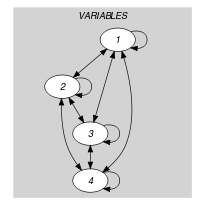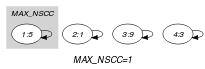(a) (b)
Automaton

Figure 5.12.6 depicts the automaton associated with the $\mathrm{𝚊𝚕𝚕𝚍𝚒𝚏𝚏𝚎𝚛𝚎𝚗𝚝}$ constraint. To each item of the collection $\mathrm{𝚅𝙰𝚁𝙸𝙰𝙱𝙻𝙴𝚂}$ corresponds a signature variable ${𝚂}_{i}$ that is equal to 1. The automaton counts the number of occurrences of each value and finally imposes that each value is taken at most one time.

##### Figure 5.12.6. Automaton of the $\mathrm{𝚊𝚕𝚕𝚍𝚒𝚏𝚏𝚎𝚛𝚎𝚗𝚝}$ constraint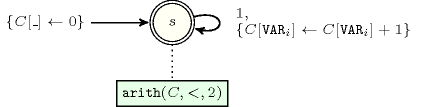Quiz

$\mathrm{𝚊𝚕𝚕𝚍𝚒𝚏𝚏𝚎𝚛𝚎𝚗𝚝}$: checking whether a ground instance holds or not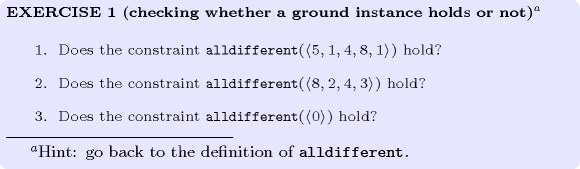$\mathrm{𝚊𝚕𝚕𝚍𝚒𝚏𝚏𝚎𝚛𝚎𝚗𝚝}$: finding all solutions$\mathrm{𝚊𝚕𝚕𝚍𝚒𝚏𝚏𝚎𝚛𝚎𝚗𝚝}$: finding all solutions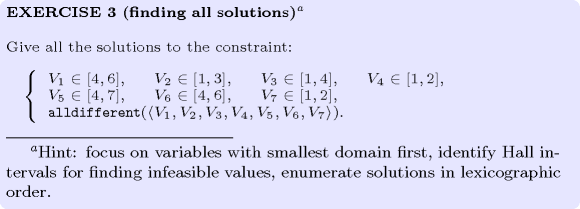$\mathrm{𝚊𝚕𝚕𝚍𝚒𝚏𝚏𝚎𝚛𝚎𝚗𝚝}$: finding all solutions$\mathrm{𝚊𝚕𝚕𝚍𝚒𝚏𝚏𝚎𝚛𝚎𝚗𝚝}$: identifying infeasible values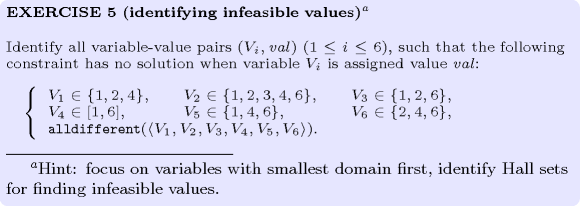$\mathrm{𝚊𝚕𝚕𝚍𝚒𝚏𝚏𝚎𝚛𝚎𝚗𝚝}$: identifying infeasible values and counting$\mathrm{𝚊𝚕𝚕𝚍𝚒𝚏𝚏𝚎𝚛𝚎𝚗𝚝}$: variable-based degree of violation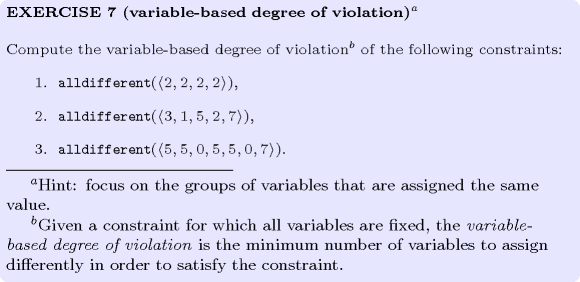$\mathrm{𝚊𝚕𝚕𝚍𝚒𝚏𝚏𝚎𝚛𝚎𝚗𝚝}$: preventing conflict around the table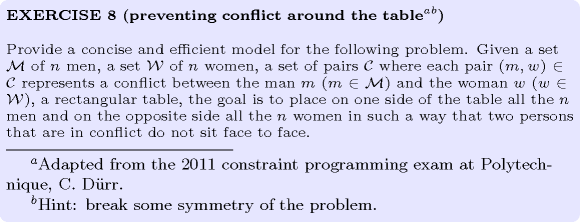$\mathrm{𝚊𝚕𝚕𝚍𝚒𝚏𝚏𝚎𝚛𝚎𝚗𝚝}$: identifying equalities from a clique of disequalities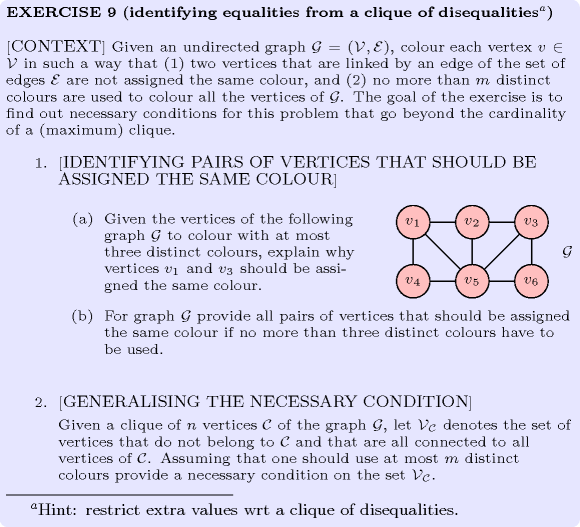$\mathrm{𝚊𝚕𝚕𝚍𝚒𝚏𝚏𝚎𝚛𝚎𝚗𝚝}$: (8-queens) unfeasibility of a partial solution